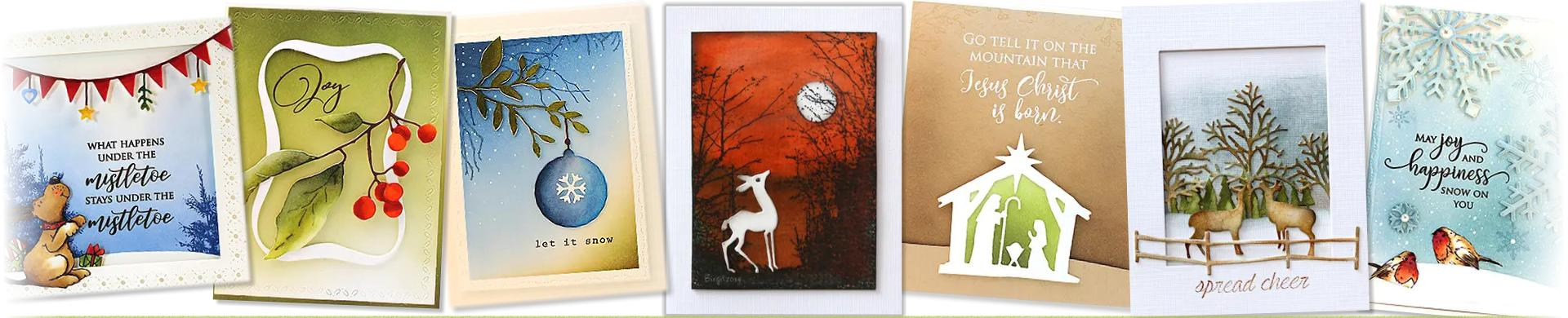# Penny Black Cutting Dies - Christmas & WinterElegant cutting dies from Penny Black for your Christmas and Winter card making.
• Brand
1. \$14.90
2. \$12.92
3. \$12.92
4. \$11.92
5. \$14.90
6. \$13.91
7. \$18.88
8. \$13.91
9. \$12.92
10. \$12.92
11. \$10.92
12. \$14.90
13. \$12.92
14. \$11.92
15. \$7.94
16. \$8.94
17. \$10.92
18. \$10.92
19. \$13.91
20. \$14.90
21. \$13.91
22. \$19.88
23. \$17.88
24. \$12.92
25. \$14.90
26. \$12.92
27. \$18.88
28. \$19.88
29. \$22.86
30. \$24.85
31. \$14.90
32. \$12.92
33. \$12.92
34. \$11.92
35. \$13.91
36. \$12.92
37. \$9.43
38. \$11.92
39. \$11.92
40. \$18.88
41. \$10.92
42. \$15.90
43. \$12.92
44. \$15.90
45. \$16.89
46. \$10.92
47. \$19.88
48. \$12.92
49. \$14.90
50. \$21.87
51. \$11.92
52. \$15.90
53. \$21.87
54. \$13.91
55. \$12.92
56. \$16.89
57. \$16.89
58. \$11.92
59. \$18.88
60. \$15.90
61. \$11.92
62. \$23.85
63. \$8.94
64. \$14.90
65. \$14.90
66. \$24.85
67. \$15.90
68. \$14.90
69. \$18.88
70. \$14.90
71. \$17.88
72. \$11.92
73. \$15.90
74. \$15.90
75. \$14.90
76. \$11.92
77. \$18.88
78. \$21.87
79. \$14.90
80. \$14.90
81. \$12.92
82. \$18.88
83. \$15.90
84. \$10.92
85. \$19.88
86. \$9.93
87. \$12.92
88. \$12.92
89. \$11.92
90. \$15.90
91. \$9.93
92. \$15.90 \$15.10
93. \$7.94 \$7.55
94. \$7.94 \$7.55
95. \$17.88 \$16.99
96. \$15.90 \$15.10